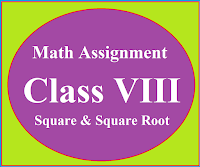### Math Assignment Class VIII | Square & Square RootMath Assignment  Class VIII | Square & Square Root Download or Print free  assignment with answer key  for   Class  8 Squares and  Square Roots.   Important and extra questions that cover all topics of square and square root and is useful and helpful for the students. Math Assignment  Class VIII | Square & Square Root LEVEL -1

### Lesson Plan Math Class 8 (Ch-2) | Cube & Cube Roots

E- LESSON PLAN   SUBJECT MATHEMATICS    CLASS- 8

Lesson Plan for CBSE mathematics class 8 Cube and Cube Roots, Step by step teaching strategy for mathematics teachers. Perfect lesson plan which makes the teaching learning process perfect

## E-LESSON PLAN MATHEMATICSCLASS-VIIICHAPTER-2 CUBE & CUBE ROOTS

 NAME OF THE TEACHER DINESH KUMAR CLASS VIII CHAPTER 02 SUBJECT MATHEMATICS TOPIC CUBE & CUBE ROOTS DURATION : 10 Class Meetings

## Materials:

Whiteboard and markers, Calculator Worksheets with problems related to squares and square roots. Real-life examples related to cube and cube roots.

## Learning Objective:

• To introduce the concept of cube & cube roots to students.
• To teach students how to calculate the cube and cube root of a number.
• To help students understand the practical applications of cubes and cube roots.
• ## Learning Outcomes

By the end of this lesson, students will be able to:

• Define cube and cube root.
• Find the cube of a given number.
• Find the cube root of a given number.
• Solve problems related to cube and cube root.

## RESOURCES

NCERT Text Book,
A Text Book of DAV Board
Resource Material :
Worksheets , E-content, Basics and formulas from (cbsemathematics.com)

## KEY WORDS:

Cube, Cube root, Factorization

## CONTENT OF THE TOPIC

Concepts and important facts related to the cube and cube roots.
• Cube of odd numbers is odd and cube of even numbers is even.
• Cube of negative numbers is negative.
• Factorization method of finding the cube root of a number.
• Method of estimation of finding the cube root of a number.
LEARNING ACTIVITIES:

## PROCEDURE

Introduction: Start the lesson by asking students if they have heard the terms "cube" and "cube root" before. Then, define what cube and cube root are:

Cube: The cube of a number is the number raised to the power of three.

For example, the cube of 2 is  2 x 2 x 2 = 8.

Cube Root: The cube root of a number is the number that when cubed, gives the original number.

For example, the cube root of 8 is 2 because 2 x 2 x 2 = 8.

Teaching:

Demonstrate how to find the cube of a given number. Use some examples such as:

33 = 27

53 = 125

(-2)3 = -8

Explain how to find the cube root of a given number. Use some examples such as:

Cube root of 27 = 3

Cube root of 125 = 5

Cube root of -8 = -2

Provide some practice problems for the students to solve in pairs or individually. Example problems may include:

a) Find the cube of 7

b) Find the cube root of 64

c) Find the cube of -4

d) Find the cube root of -125

Once students have completed the practice problems, go over the answers as a class.

## Assessment:

Have the students complete a worksheet with additional practice problems on cube and cube root. Collect the worksheets and review them to identify any areas where students may need additional instruction.

## Conclusion:

Summarize the key concepts covered in the lesson and remind students of the importance of understanding cube and cube root in mathematics. Encourage them to practice these skills further to strengthen their understanding of the topic.